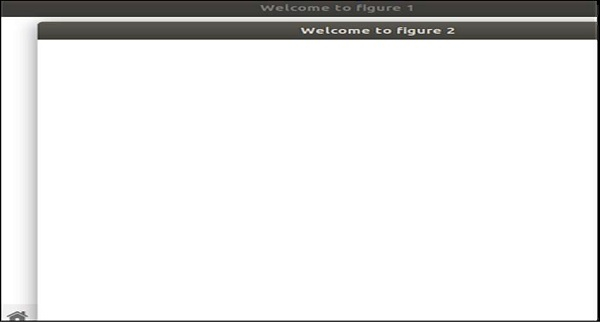How to set the current figure in Matplotlib?

MatplotlibServer Side ProgrammingProgramming

Using the figure() method, we can set the current figure.

Steps

• Create a new figure, or activate an existing figure, with the window title “Welcome to figure 1”.

• Create a new figure, or activate an existing figure, with the window title “Welcome to figure 2”.

• Using plt.show(), show the figures.

Example

from matplotlib import pyplot as plt

plt.figure("Welcome to figure 1")
plt.figure("Welcome to figure 2")    # Active Figure

plt.show()

Output